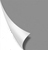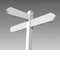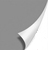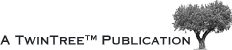#### 01-03 The Magnetic Resonance Signal and its Componentsagnetic resonance signals are time-varying electric currents or voltages. They come in waves which are induced by an oscillating magnetic field. The form of the wave reflects the information in the signal.

Basically, magnetic resonance signals are sine and cosine waves which are described and defined by the three factors: amplitude, frequency, and phase (Figure 01-05).

Amplitude is also called signal strength. It reflects the final brightness of a picture ele­ment of a magnetic resonance image. Frequency and phase de­ter­mine shape and spa­tial detail in an MR image. Amplitude is the difference bet­ween the peak of the cur­ve and zero. Its usual unit is volt (V), in our context usually mV.

Figure 01-05:
Amplitude, frequency, and phase (t = time).

Frequency is the number of complete cycles per second. It is measured in Hertz (Hz; 1 Hz = 1 cycle per second). In magnetic resonance imaging we usually deal with kilo­hertz (1 kHz = 1,000 Hz) or megahertz (MHz = 1,000,000 Hz).

Phase specifies the initial amplitude of a wave. Phase can only be compared for waves with the same frequency. Phase differences are expressed in degrees. One complete cy­cle equals 360° (Figure 01-06).

Figure 01-06:
Phase (t = time).

If there are two waves with the same frequency which are shifted from each other, there is a phase shift or phase offset (Figure 01-07).

Figure 01-07:
Phase shift. Two sine waves are shifted 90° and 180° from each other.